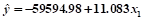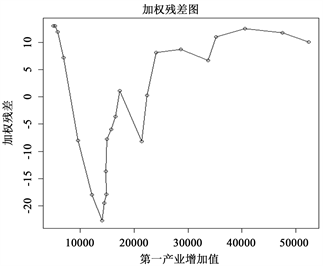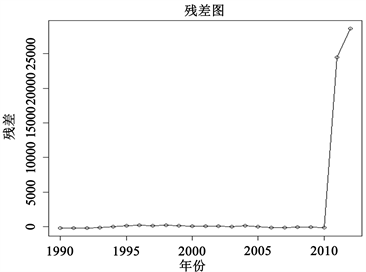# 我国GDP与第一、二、三产业的相关关系分析An Analysis of the Correlation between GDP and the Primary Industry, Secondary Industry and Tertiary Industry in China

DOI: 10.12677/SA.2020.92018, PDF, HTML, XML, 下载: 158  浏览: 698

Abstract: According to the data about primary, secondary and tertiary industry in 1990-2012 from big data platform, this paper sets up a simple linear regression model () between GDP and the adding value of the primary industry by using the statistical software R and SPSS. Then the test of Correlation Coefficient shows that the model has heteroscedasticity and autocorrelation which were eliminated by “one variable weighted least square estimation” and FDM. So the model is improved. Then this paper uses the GDP and the first, the second, the third industry to establish the multiple linear regression model. Due to the significance of the model is not ideal, this paper uses the stepwise regression method to screen the independent variables and finally establishes the multiple linear regression model () between GDP and the secondary industry and tertiary industry. Finally, based on the established model, we can use the future changes in the primary, secondary and tertiary industries to reasonably forecast the GDP growth rate.

1. 引言

2. 一元回归模型的建立

2.1. 普通最小二乘估计

2.1.1. 普通最小二乘回归方程求解

${\stackrel{^}{\beta }}_{0}=\stackrel{¯}{y}-{\stackrel{^}{\beta }}_{1}\stackrel{¯}{x}$ (3-1)

${\stackrel{^}{\beta }}_{1}=\frac{\underset{i=1}{\overset{n}{\sum }}\left({x}_{i}-\stackrel{¯}{x}\right)\left({y}_{i}-\stackrel{¯}{y}\right)}{\underset{i=1}{\overset{n}{\sum }}{\left({x}_{i}-\stackrel{¯}{x}\right)}^{2}}$ (3-2)

(公式里 ${x}_{i}$ 对应第一产业 ${x}_{1}$ 的相应值， ${y}_{i}$ 对应GDP的相应值)。Table 1. The output of ordinary least squares regression to primary sector of the economy and GDP

a. 因变量：GDP。

$\stackrel{^}{y}=-59594.98+11.083{x}_{1}$ (3-3)

2.1.2. 残差分析Figure 1. Residuals for different primary sector of the economy

2.2. 一元加权最小二乘估计

2.2.1. 异方差性的检验Table 2. Rank correlation coefficient output

**.在0.01级别(双尾)，相关性显著。

2.2.2. 一元加权最小二乘模型的建立

${\stackrel{^}{\beta }}_{0w}={\stackrel{¯}{y}}_{w}-{\stackrel{^}{\beta }}_{1w}{\stackrel{¯}{x}}_{w}$ (3-4)

${\stackrel{^}{\beta }}_{1w}=\frac{\underset{i=1}{\overset{n}{\sum }}{w}_{i}\left({x}_{i}-{\stackrel{¯}{x}}_{w}\right)\left({y}_{i}-{\stackrel{¯}{y}}_{w}\right)}{\underset{i=1}{\overset{n}{\sum }}{w}_{i}{\left({x}_{i}-{\stackrel{¯}{x}}_{w}\right)}^{2}}$ (3-5)

① 确定幂指数m的最优值，输出结果如表3Table 3. Weight function output

a. 选择了相应的幂进行进一步分析，这是因为，它使对数似然函数最大化；b. 因变量：GDP，源变量：第一产业。

$m=-1.5$ (3-6)

② 建立模型Table 4. UNARY weighted least square estimation output

a. 因变量：GDP；b. 加权最小平方回归–按权重加权。

$\stackrel{^}{y}=-39785.149+10.002{x}_{1}$ (3-7)Figure 2. Weighted least square estimation residual diagram

2.3. 迭代法建立模型

2.3.1. 样本相关系数的计算Table 5. Output table of the correlation coefficient between primary sector of the economy and GDP

**.在0.01级别(双尾)，相关性显著。

2.3.2. 利用差分法消除自相关性Table 6. The output of the regression model established by the difference method

a. 因变量：差分后的GDP；b. 过原点线性回归。

$\Delta y=0.181\Delta x$ (3-8)

${\stackrel{^}{y}}_{t}-{\stackrel{^}{y}}_{t-1}=0.181\left({x}_{t}-{x}_{t-1}\right)$ (3-9)

3. 多元回归模型的建立

3.1. 多元回归模型的普通最小二乘估计

3.1.1. 回归模型的建立

$\stackrel{^}{\beta }={\left({x}^{\text{T}}x\right)}^{-1}{x}^{\text{T}}y$ (4-1)Table 7. The output of the coefficient calculation of multivariate regression equation

a. 因变量：GDP。Table 8. Independent variable correlation test output

**.在0.01级别(双尾)，相关性显著。

3.1.3. 逐步回归法  选择自变量

${\alpha }_{\text{entry}}=0.1$${\alpha }_{\text{removal}}=0.15$，利用SPSS软件进行运算，输出结果见表9

Table 9. The result of the stepwise regression

a. 因变量：GDP。

$\stackrel{^}{y}=5377.346+1.216{x}_{2}+0.976{x}_{3}$ (4-2)

3.2. 残差分析

3.2.1. 残差计算与绘图Figure 3. Residuals graph of multiple linear regression

4. 评价与总结

  张怡, 熊常伟. 基于南充市生产总值(GDP)的灰色预测与因素分析[EB/OL]. http://kns.cnki.net/KXReader/Detail?TIMESTAMP=637183386499466250&DBCODE=CJFQ&TABLEName=CJFD2014&FileName=IGNE201403008&RESULT=1&SIGN=venkkEOhs%2bHgiQwGe53xKddtbr0%3d, 2019-12-12. %%%  中华人民共和国国家统计局. 中国统计年鉴: 2013[M]. 北京: 中国统计出版社, 2013.  何晓群, 刘文卿. 应用回归分析(第5版)[M]. 北京: 中国人民大学出版社, 年份.  孙玲, 张静. 基于多元线性回归的粤西地区经济发展影响因素分析[EB/OL]. http://kns.cnki.net/KXReader/Detail?TIMESTAMP=637185147123372500&DBCODE=CJFQ&TABLEName=CJFDLAST2019&FileName=DAJJ201910016&RESULT=1&SIGN=UGCJi1txT2nNKA45Ms5XROo3p1I%3d.  蔡思美. 逐步回归法的财政收入影响因素分析[EB/OL]. http://kns.cnki.net/KXReader/Detail?TIMESTAMP=637185150096966250&DBCODE=CJFD&TABLEName=CJFDLAST2019&FileName=KJSZ201914011&RESULT=1&SIGN=XdvbkRAj2erlXnUJ3PQ6hs8jxFk%3d.